# 4 Nonlinear analysis for large amplitude solitary waves

Anticipating constant profile solutions, we shall consider Eqs. (9 )-(12) in a stationary frame traveling at a constant normalized velocity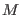(to be referred to as the Mach number), implying the transformation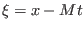. The space and time derivatives are thus replaced by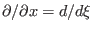and, respectively, so Eqs. (9)-(12) take the form: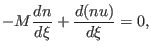(21)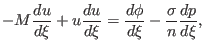(22)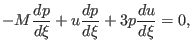(23)(24)

We assume that the equilibrium state is reached at both infinities (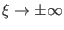). Accordingly, we integrate and apply the boundary conditions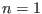,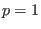,and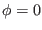at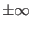. One thus obtains(25)(26)

and(27)

Combining Eqs. (25)-(27), we obtain the following biquadratic equation for the cool electron density,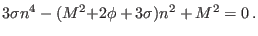(28)

The solution of Eq. (28) may be written as(29)

where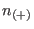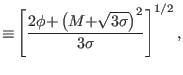(30)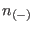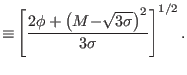(31)

From the boundary conditions,at, it follows that the negative sign must be taken in Eq. (29). Furthermore, we shall assume that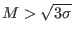, i.e., that the cool electrons are supersonic, while the hot electrons are subsonic, thus we require that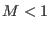.

Reality of the density variable imposes the requirement, which implies a limit on the electrostatic potential value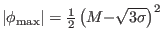associated with negative solitary structures (positive electric potentials, should they exist, satisfy the latter condition automatically, and are thus not limited).

Substituting the density expression (29)-(31) into Poisson's equation (24) and integrating, yields the pseudo-energy balance equation for a unit mass in a conservative force field, if one definesas time'' and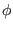as position'' variable: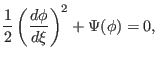(32)

where the Sagdeev pseudopotential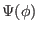is given by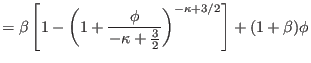(33)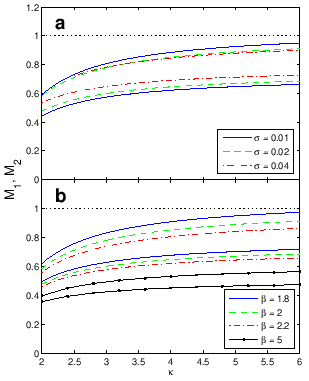Ashkbiz Danehkar
2018-03-28